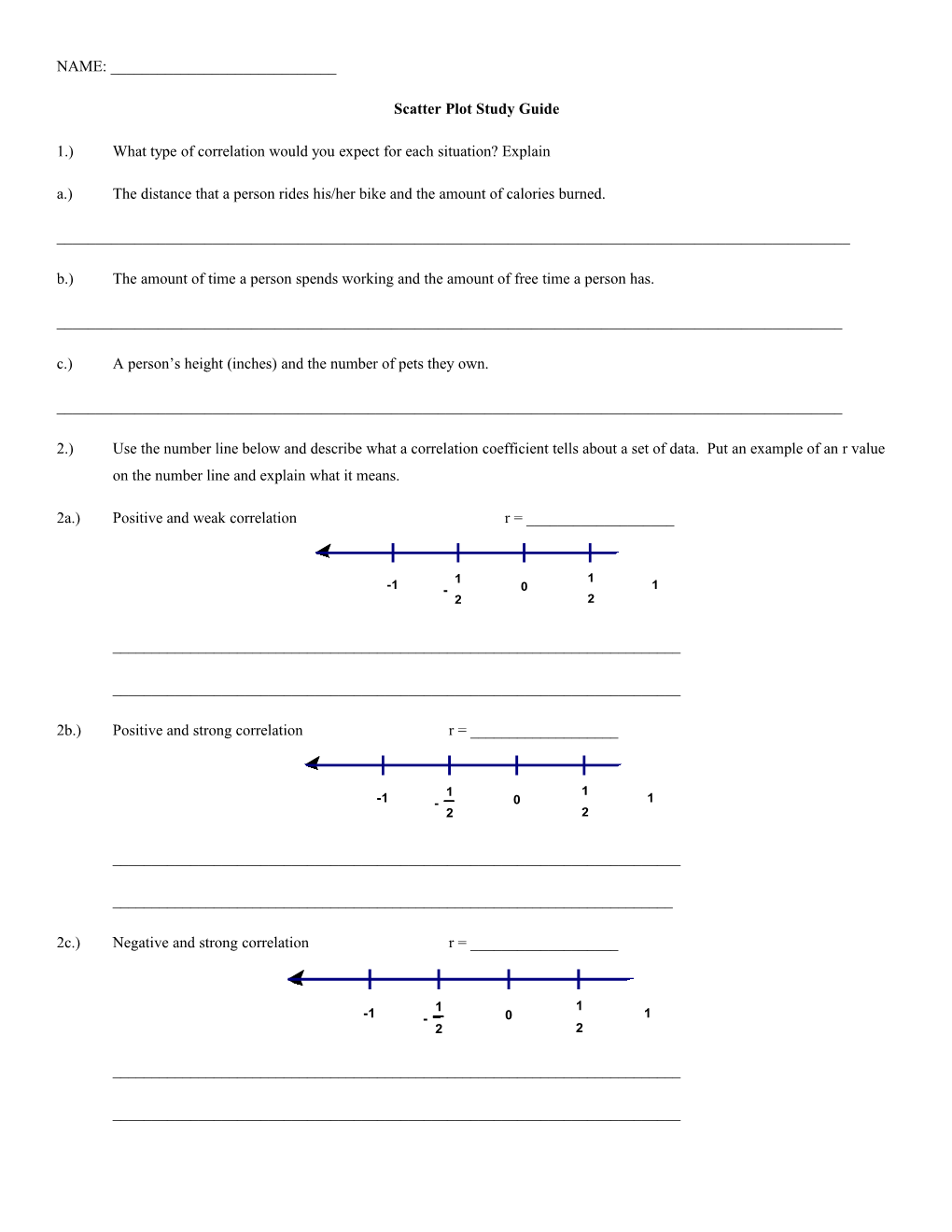# Scatter Plot Study GuideNAME: ______

Scatter Plot Study Guide

1.)What type of correlation would you expect for each situation? Explain

a.)The distance that a person rides his/her bike and the amount of calories burned.

______

b.)The amount of time a person spends working and the amount of free time a person has.

______

c.)A person’s height (inches) and the number of pets they own.

______

2.)Use the number line below and describe what a correlation coefficient tells about a set of data. Put an example of an r value on the number line and explain what it means.

2a.)Positive and weak correlationr = ______

______

______

2b.)Positive and strong correlationr = ______

______

______

2c.)Negative and strong correlationr = ______

______

______

2d.)Negative and weak correlationr = ______

______

______Use the line of best fit to answer questions 3 and 4

3.)Based on the line of best fit, predict the daily sales if the temperature was 54 degrees.

______

4.)Based on the line of best fit, predict the temperature if the daily sales was \$100.

______

Speed (mi/h) / Stopping Distances (ft)
10 / 27
15 / 44
20 / 63
25 / 85
30 / 109
35 / 136
40 / 164
45 / 196

Use the table of values to answer questions 5 – 7.

5.)Enter this data into your calculator.

What is the slope?______

What is the y-intercept?______

What is the correlation coefficient? ______

What is the best fit line?______

6.)Using your best fit line for this data, predict what the stopping distance is after 55 miles per hour.

______

7.)Using your best fit line for this data, predict the speed in miles per hour it would take for the stopping distances to be 250 feet.

______

Use the table of values to answer questions 8 – 10.

Minutes Using
Cell Phone / Battery Charge
Percentage
0 / 100
10 / 95
20 / 88
30 / 85
40 / 79
50 / 72
60 / 68

8.)Enter this data into your calculator.

What is the slope?______

What is the y-intercept? ______

What is the correlation coefficient? ______

What is the best fit line? ______

9.)Use your line of best fit to determine how many minutes you will use when the batter charge percentage is at 25% .

______

10.)Use your line of best fit to determine how much battery life you have after using your phone for 75 minutes.

______

Use your calculator to make a scatter plot of the given data. Then answer the following questions.

Judy is a waitress at Applebee’s. She works several days in a two week period. The table shows the number of hours she works and the amount she earns in tips each day.

Hours / 4 / 5 / 4 / 7 / 6 / 5
Tips / \$27 / \$30 / \$23 / \$64 / \$49 / \$55

What is the slope? ______

What is the y-intercept?______

What is the correlation coefficient?______

What is the best fit line?______

11. Using your best fit line find the amount of tips if you worked 10 hours. Show your work below.

______

12. Using your best fit line find when you will make about 300 dollars in tips. Show your work below.

______